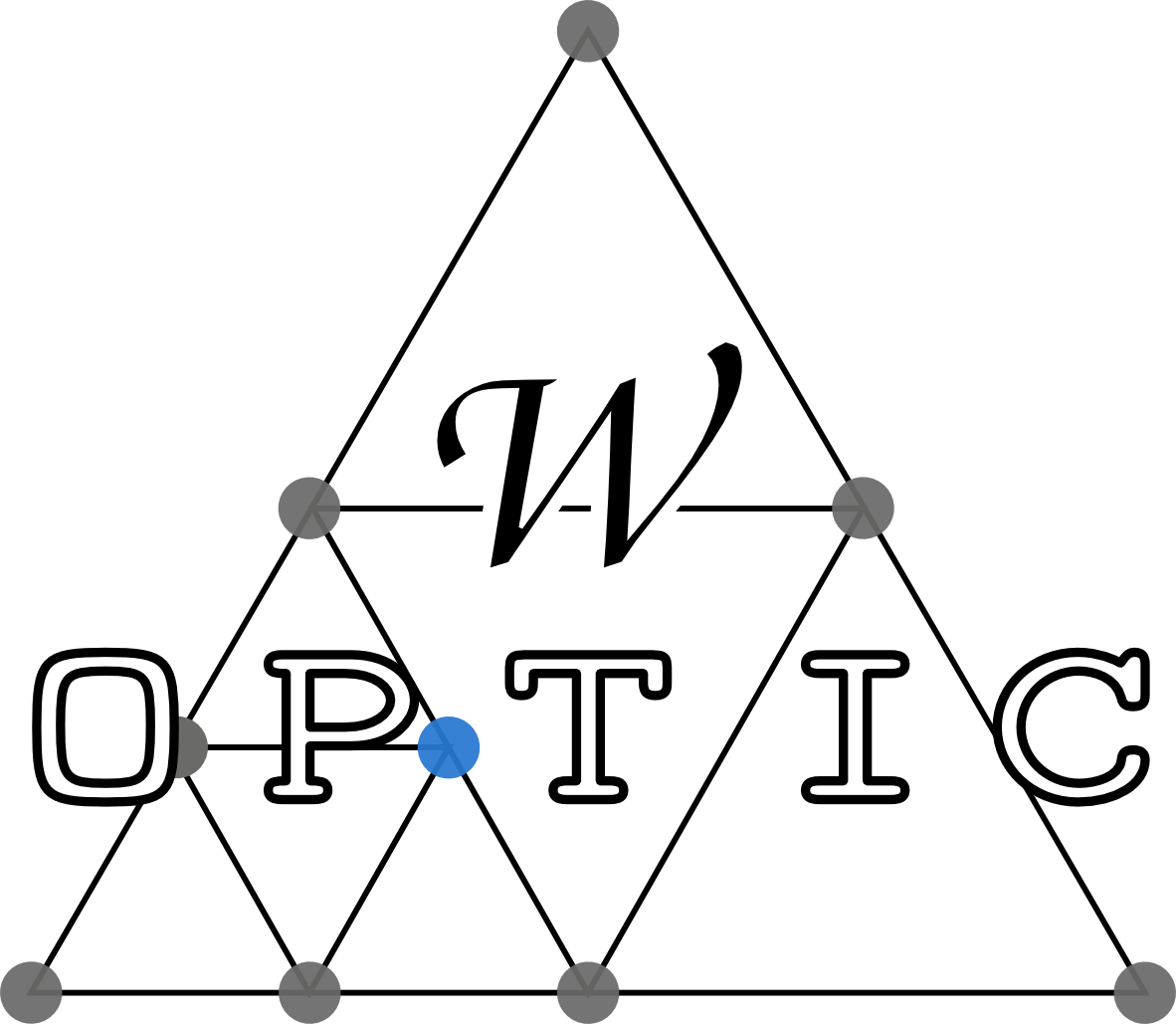## optical conductivity with Wannier functions and adaptive k-mesh refinement — CPC 202, 1 (2016)calculates the optical conductivity of interacting systems in a maximally-localized Wannier basis from the expression \begin{equation*} \label{eq:optcond} \DeclareMathOperator{\tr}{tr} \newcommand\dd {\text{d}} \newcommand\ii {\text{i}} \newcommand\ee {\text{e}} \newcommand\ii {\text{i}} σ_{αβ}(Ω) = \tfrac{e^2}{(2π)^2} \hbar \int\!\dd^3 k \int\!\dd ω\mathop{} w(ω; Ω) \tr\left[A(k,ω)\,V^α(k)\,A(k,ω+Ω)\,V^β(k)\right] \end{equation*} where $σ^{αβ}(Ω)$ is the $(α,β)$ element of the optical conductivity tensor ($α,β \in \{x,y,z\}$) at external frequency $Ω$, $w(ω; Ω) = [f(ω)-f(ω+Ω)]/Ω$ is a weight in terms of the Fermi functions $f$, $A= \ii(G-G^\dagger)/(2 π)$ the generalized spectral function, and $V^α$ the group velocity in direction $α$ , , . The numerical bottleneck in evaluating $σ^{αβ}$ is the k-summation, since usually many k-points are required to obtain converged results.

is built on top of Wien2k , wien2wannier , and Wannier90 . It consists of two main programs: woptic_main, which calculates the optical conductivity, and refine_tetra, where the k-mesh is refined; as well as several smaller support programs. The individual programs are normally called by means of the driver script woptic.

### Features

• Many-body calculations can incorporate a many-body self-energy $Σ(ω)$, e.g. from DMFT. “Uncorrelated” bands not treated in DMFT can be included as an outer window.
• Adaptive integration The contributions to $σ^{αβ}$ are often sharply peaked in k-space. employs an adaptive tetrahedral grid for efficient Brillouin-zone integration.
• Full dipole matrix elements Often, the band velocities $V^α$ are approximated by k-derivatives of the bandstructure. uses the full matrix elements as calculated by Wien2k's optic module .
• ### References

The canonical reference for is , which should be cited in any publications resulting from the use of the code. Two PhD theses are concerned with , . They explain the formalism and the workings of the code in greater detail. The chapter relating to from the latter thesis is available here.

E. Assmann, P. Wissgott, J. Kuneš, A. Toschi, P. Blaha, and K. Held. woptic: optical conductivity with Wannier functions and adaptive k-mesh refinement. Comput. Phys. Commun. 202, 1 (2016); arXiv:1507.04881.

E. Assmann. Spectral properties of strongly correlated materials. PhD Thesis, TU Wien, Vienna (2015). Download the chapter.

P. Blaha, K. Schwarz, G.K.H. Madsen, D. Kvasnicka, and J. Luitz. Wien2k, An Augmented Plane Wave + Local Orbitals Program for Calculating Crystal Properties. Techn. Universität Wien, Vienna, (2001). http://www.wien2k.at.

J. Kuneš, R. Arita, P. Wissgott, A.Toschi, H. Ikeda, and K. Held. Wien2wannier: From linearized augmented plane waves to maximally localized Wannier functions. Comput. Phys. Commun. 181, 1888 (2010); arXiv:1004.3934.

A.A. Mostofi, J.R. Yates, Y.-S. Lee, I. Souza, D. Vanderbilt, and N. Marzari. Wannier90: A tool for obtaining maximally-localized Wannier functions. Comput. Phys. Commun. 178, 685 (2008); arXiv:0708.0650

J.M. Tomczak and S. Biermann. Optical properties of correlated materials: Generalized Peierls approach and its application to VO₂. Phys. Rev. B 80, 085117 (2009).

J.M. Tomczak. Spectral and optical properties of correlated materials. PhD Thesis, École Polytechnique, Paris (2007).

P. Wissgott, J. Kuneš, A. Toschi, and K. Held. Dipole matrix element approach versus Peierls approximation for optical conductivity. Phys. Rev. B 85, 205133 (2012).

P. Wissgott. Transport properties of correlated materials from first principles. PhD Thesis, TU Wien, Vienna (2012).

### Acknowledgements

was developed at Vienna and Graz Universities of Technology with support from the European Research Council through grant agreement no. 306447 and the Austrian science fund (FWF) through start project Y746.